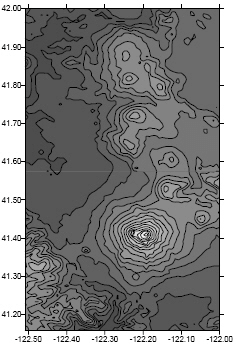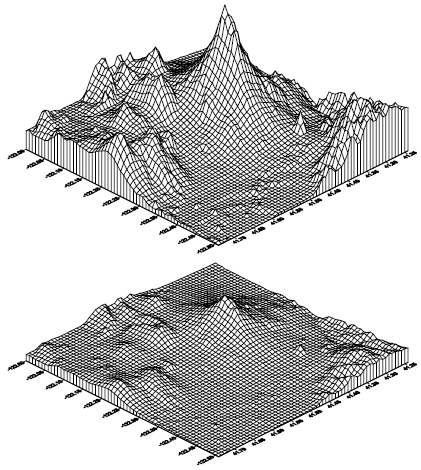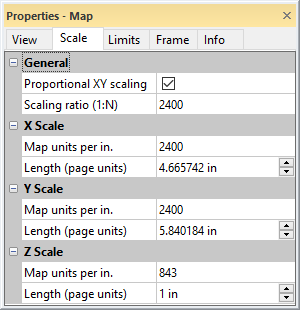# Scale

The scale controls the size of a map or profile on the printed page. This is accomplished by defining a correspondence between lengths on the map (in map units) and lengths on the printed page (in page units). The map scale is specified using the Scale page in the map properties. The scale for the X axis and the scale for the Y axis can be set in unison (proportionally), or they can be set independently. On three-dimensional maps (i.e. wireframes and surface maps), the scale for the Z axis can also be set.

Axes are part of the map on which they are drawn. Therefore, when the scale is redefined, the axes are modified accordingly. Because Surfer calculates reasonable tick spacing based on the map lengths and map limits, redefining the scales can result in different tick spacing along the axis.

## Default Scale

By default, map scales are defined proportionally in the X and Y dimensions, with the longest side measuring six inches on the printed page. For example, consider a map extending 60 map units in the X dimension and 40 map units in the Y dimension. Since the extent in the X dimension is longer than the extent in the Y dimension, the map is drawn six inches wide. The resulting scale is 10 map units per inch. The scale in the Y dimension is also 10 map units per inch, so the map is drawn four inches in height.This map scale was customized. The Y dimension covers a greater extent than the X dimension in this map. By default, the Y axis would be six inches in length, and the X axis is proportionally shorter.These are two 3D wireframe maps of the same grid, each using a different Z scale. The Z scale is exaggerated in the top view. Use the Scale page to change the length of the Z axis.

On three-dimensional maps the default scale in the Z dimension is defined such that the vertical extent of the map is one-fourth the maximum horizontal extent.

The default scales are a function of the map limits. Map limits are determined by the file used to create the map, or they can be defined using Limits page.

## Scale Page

The Scale page is located in the map properties.Specify the relative X, Y, and Z map scaling on the Scale page in the Properties window.

### Proportional XY Scaling

The Proportional XY scaling check box ensures that the X and Y dimensions are scaled equally. When the box is checked, a change in either the X or Y dimension is reflected in the other dimension by a proportional amount. To scale the axes independently, uncheck the box.

Sizing a map with its selection handles rescales the map internally. To return to the proper scale, use the Home | Selection | Transform command to clear the transform, and then use the Scale page to scale the map.

When Proportional XY scaling is checked, the Scaling ratio (1:N) option is available. The Scaling ratio (1:N) property is hidden when Proportional XY scaling is not checked.

### Scaling Ratio

Specify the X and Y map scale representative fraction by typing the desired value in the Scaling ratio (1:N) property field. The Proportional XY scaling check box must be checked to scale maps with by representative fraction. Press ENTER or click a different property to update the scaling values. Changing the Scaling ratio (1:N) automatically updates the X Scale and Y Scale sections Map units per in (cm) and Length (page units) property values.

The representative fraction scaling method shows the map scale as a ratio of distance on the map (1) to distance on the ground (N). For example, a representative fraction of 1:100000 means one centimeter on the map represents 100,000cm on the ground (1000m or 1km). Similarly on a 1:100000 scale map, 1 inch on the map represents 100,000 inches on the ground (8333.3ft or 1.578 miles).

When perspective, tilt, and rotation are applied to a map view, the ratio is honored for the center of the map in the X direction. The ratio may not be valid for other regions of the map, depending on the View page settings.

### X, Y, and Z Scale

The X Scale, Y Scale, and Z Scale sections specify the scale along the indicated axis. Each axis can be scaled independently by clearing the Proportional XY scaling check box. When the Proportional XY scaling box is checked, any changes made to the scale in the X or Y dimension is automatically reflected in the other dimension. Z scaling is always independent of the X and Y scaling. Similarly, any change in the X or Y scale values automatically updates the Scaling ratio (1:N) value when Proportional XY scaling is checked.

#### Map Units Per In. (cm.)

The Map units per in. (cm.) option sets the number of map units per page unit in the associated dimension. For example, to draw a map at a scale of 1000 map units per inch, type the value 1000 into the box. The Length (page units) value is automatically updated to reflect the change. For example, if the map is 8000 units in the dimension you are setting, the map is 8 inches long in that dimension.

#### Length

The Length (page units) option sets the length of the map in the X, Y, or Z dimension. When the Length (page units) value is changed, the Map units per In. (cm.) box is automatically updated to reflect the change.